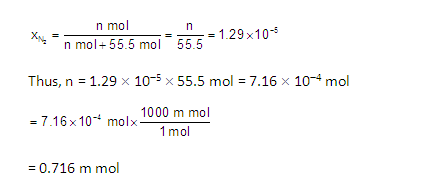Welcome to Chem Zipper.com......: If N2 gas is bubbled through water at 293 K, how many millimoles of N2 gas would dissolve in 1 litre of water. Assume that N­2 exerts a partial pressure of 0.987 bar. Given that Henry’s law constant for N2 at 293 K is 76.84 kbar.

## Thursday, September 29, 2022

### If N2 gas is bubbled through water at 293 K, how many millimoles of N2 gas would dissolve in 1 litre of water. Assume that N­2 exerts a partial pressure of 0.987 bar. Given that Henry’s law constant for N2 at 293 K is 76.84 kbar.

The solubility of gas is related to its mole fraction in the aqueous solution. The mole fraction of the gas in the solution is calculated by applying Henry’s law. Thus,
As 1litre water contains 55.5 mol of it, therefore, if n represents number of moles of N2 in solution,Related Questions: